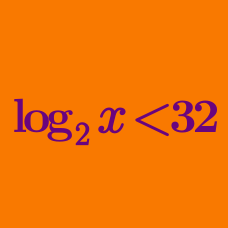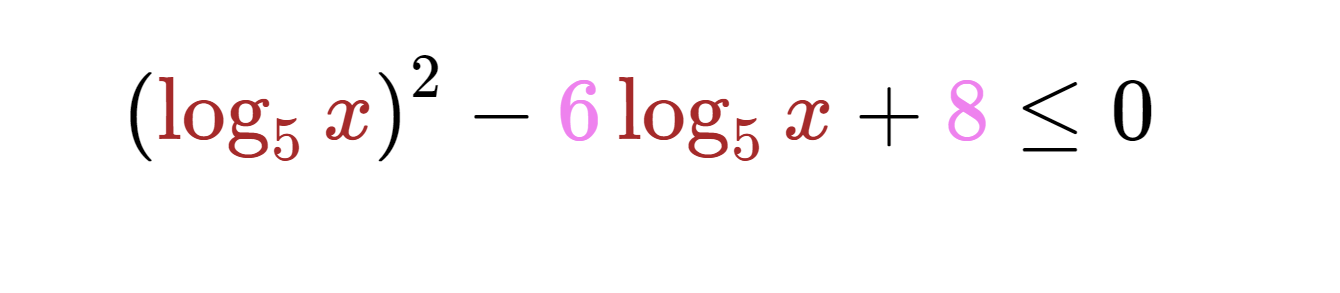Algebra

# Logarithmic Inequalities - Problem SolvingWhat is the range of $x$ that satisfies the above logarithmic inequality?

The sound intensity level (measured in decibels) is given by the formula: $L_I=10\log\left(\frac{I}{I_0}\right)\text{ (dB)},$ where $I$ denotes sound intensity and $I_0$ is the reference sound intensity.

An individual is diagnosed as moderate hearing loss when his/her threshold of sound perception is greater than 40 dB and less than or equal to 55 dB. Given that $I_0=1\text{ pW/m}^2,$ what is the range of the threshold of sound intensity $x$ that a person with moderate hearing loss can perceive?

Details and Assumptions:
- The sound intensity level of a normal conversation is about 50~60 dB, and that of a quiet library is about 40 dB.
- Assume that $\sqrt{10}\approx3.$

$A, B$ and $C$ are positive integers satisfying $A \geq 2, B \geq 4, C \geq 8$. What is the minimum integer value of

$\log_A (BC) + \log_B (CA) + \log_C (AB) ?$

Details and assumptions

The minimum integer value of a set, is the smallest integer that appears in the set. As an explicit example, the minimum integer value of the set of numbers $x$ satisfying $x \geq 12.3$ is 13.

If one root of the following quadratic equation $x^2-2x\log_{9}a+4\log_{9}a=0$ is positive and the other root is negative, what is the range of $a?$

If the range of $x$ that satisfies the inequality ${4}^4 {7}^4 x^3 > x^{\log_{28}x}$ is $\alpha < x < \beta,$ what is the value of $\alpha\beta ?$

×